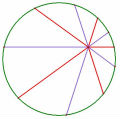# a+b+c=3,a^3+b^3+c^3=3,求a^95+b^95+c^95=?

## a+b+c=3,a^3+b^3+c^3=3,求a^95+b^95+c^95=?

$$a,b,c\in Z$$，$$a+b+c=3,a^3+b^3+c^3=3$$，求$$a^{95}+b^{95}+c^{95}=$$？

TOP

 Isaac發私訊 加為好友 目前離線 2# 大 中 小 發表於 2009-6-7 19:12  只看該作者 換個蠢方式 $$\left\{\begin{matrix} a+b+c=3 a^{3}+b^{3}+c^{3}=3\\ a+b=3-c a^{3}+b^{3}=3-c^{3} \end{matrix}\right.$$ 上式代入 $$a^{3}+b^{3}=(a+b)^{3}-3ab(a+b)$$ $$3-c^{3}=(3-c)^{3}-3ab(3-c)$$ $$(3-c)((3-c)^{2}-3ab)-3+c^{3}=0$$ 整理可得 $$8=(3-c)(3c+ab)$$ $$\left\{\begin{matrix} 3-c=1 3c+ab=8 \\ 3-c=-1 3c+ab=-8 \\ 3-c=2 3c+ab=4 \\ 3-c=-2 3c+ab=-4 \\ 3-c=4 3c+ab=2 \\ 3-c=-4 3c+ab=-2 \\ 3-c=8 3c+ab=1 \\ 3-c=-8 3c+ab=-1 \end{matrix}\right.$$ $$\left\{\begin{matrix} c=2 ab=2 \\ c=4 ab=-20 \\ c=1 ab=1 \\ c=5 ab=-19 \\ c=-1 ab=5 \\ c=7 ab=-23 \\ c=-5 ab=16 \\ c=11 ab=-34 \end{matrix}\right.$$ 列出各種組合的解，然後驗證 怎麼工程這麼浩大 有沒有便利一點的呢? (感覺這樣很蠢) 自己要多加強~~拍謝 也謝謝指導 [ 本帖最後由 Isaac 於 2009-6-8 09:06 AM 編輯 ] UID264 帖子38 閱讀權限10 上線時間126 小時 註冊時間2009-5-22 最後登入2009-10-2  查看詳細資料 TOP
 老王 老王發私訊 加為好友 目前離線 3# 大 中 小 發表於 2009-6-7 21:04  只看該作者 不止 還有 $$a=b=4,c=-5$$ 好吧 我得翻箱倒櫃看看能否找到當時印下來的題目 當年以為題目會一直掛在網站上 沒想到三天就拿掉了 名豈文章著官應老病休飄飄何所似Essential isolated singularity UID261 帖子308 閱讀權限10 上線時間943 小時 註冊時間2009-5-14 最後登入2014-3-17  查看詳細資料 TOP
 ksjeng發私訊 加為好友 目前離線 4# 大 中 小 發表於 2009-6-7 22:32  只看該作者 老師謝謝你喔 UID174 帖子206 閱讀權限10 上線時間109 小時 註冊時間2008-9-6 最後登入2018-3-20  查看詳細資料 TOP

TOP

﻿